```        []
```
(Showing Draft Content)

# Add a Measure or a Calculated Column

Measures and calculated columns are used to perform complex arithmetic calculations on your data. They enable you to build new data from the existing data, available in the cached model, with the help of analytical expressions. An analytical expression consists of functions, operators, functions, operators, and value references, which are evaluated as a formula to generate results.

In general, measures are used to perform aggregate operations on an entity such as total, count, average, percentage, and more. While calculated columns are used to perform row-by-row calculations on the target entity. Note that measures and calculated columns can only reference attributes in analytical expressions. To learn more about measures and calculated columns, please see this article.

Consider a sales entity, namely FactOnlineSales that contains information related to sales, discounts, and returns. We can use this entity to calculate the gross profit for online sales using the below analytical expression.

``````SUMX (
FactOnlineSales,
FactOnlineSales[SalesAmount] - FactOnlineSales[DiscountAmount] - FactOnlineSales[ReturnAmount] - FactOnlineSales[UnitCost] * ( FactOnlineSales[SalesQuantity] - FactOnlineSales[ReturnQuantity] )
)``````

Follow the below steps to add a measure with the above expression to the FactOnlineSales entity.

1. From the Entities panel on the left, select the FactOnlineSales entity.

2. Click the More button and then select the Add Measure option from the list.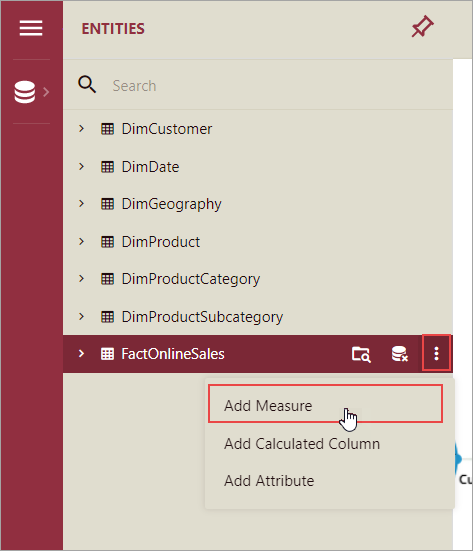3. In the Expression Editor, enter the expression for the gross profit.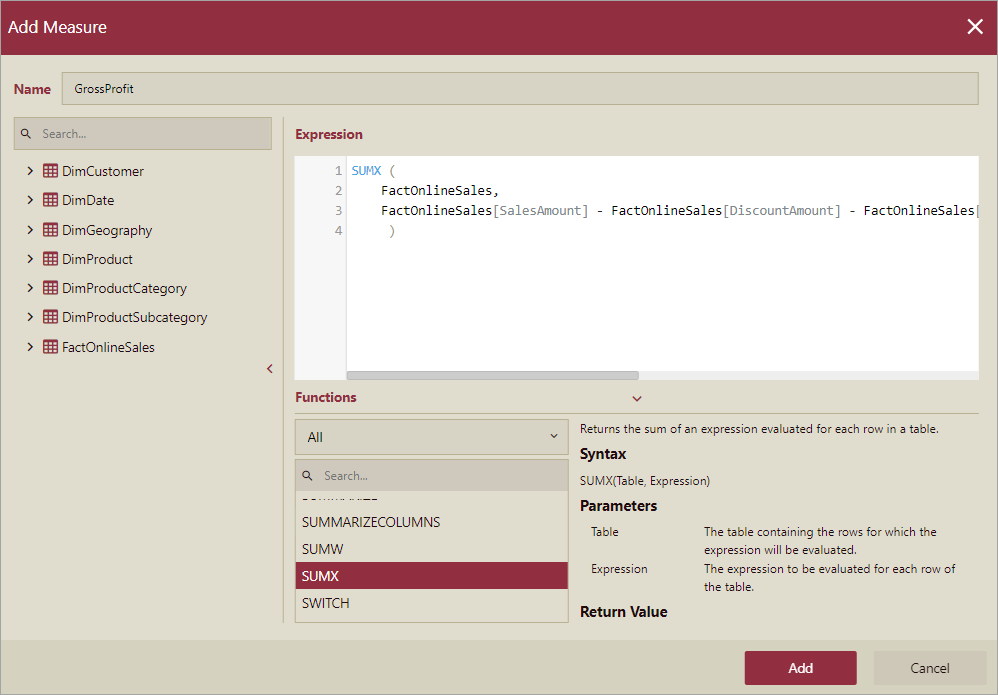4. Then, click the Add button.

The measure now appears under the chosen entity in the Entities panel along with its other attributes.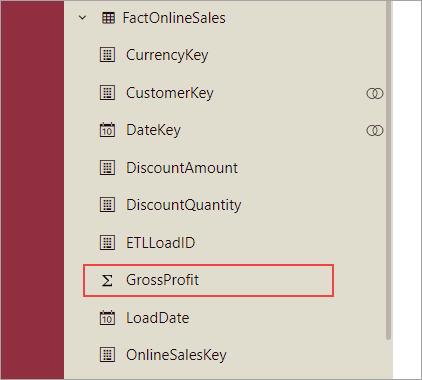### To Add a Calculated Column

Let's say, you want to use the DimCustomer entity to divide the customers into groups based on their yearly income. To achieve this, use the following expression for the calculated column, which will categorize the customers into Low, Medium, and High according to their yearly income.

``````SWITCH (
TRUE,
DimCustomer[YearlyIncome] < 50000, "Low",
DimCustomer[YearlyIncome] >= 50000 && DimCustomer[YearlyIncome] < 100000, "Medium",
DimCustomer[YearlyIncome] >= 100000, "High"
)``````

Follow the below steps to add a calculated column with the above expression to the DimCustomer entity.

1. From the Entities panel on the left, select the DimCustomer entity.

2. Click the More button and then select the Add Calculated Column option from the list.3. In the Expression Editor, enter the expression for the income range.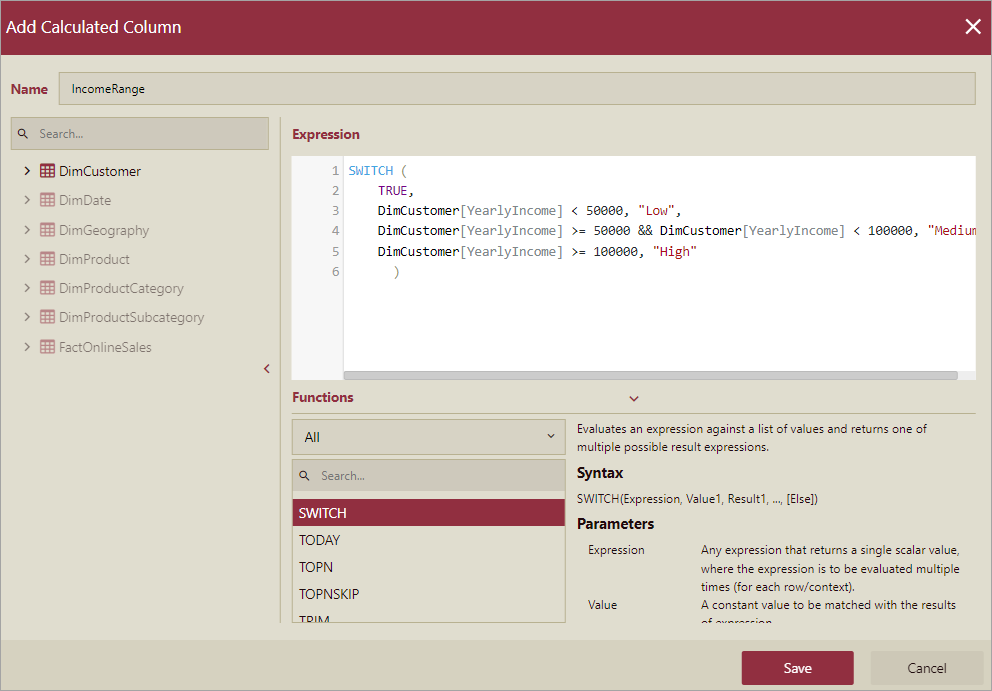4. Then, click the Add button.

The calculated column now appears under the chosen entity in the Entities panel along with its other attributes.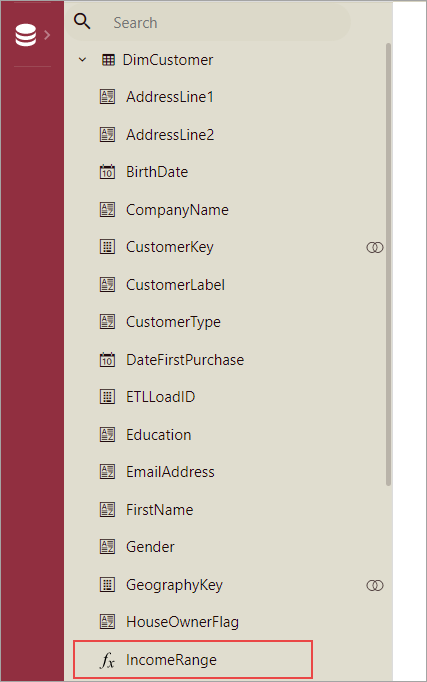### To Edit a Measure or a Calculated Column

Follow the below steps to edit a measure or a calculated column in an entity.

1. From the Entities panel, select the entity which contains the measure or calculated column you want to modify.

2. Then, choose the measure or calculated column.

3. Click the Edit button next to the chosen measure or calculated column.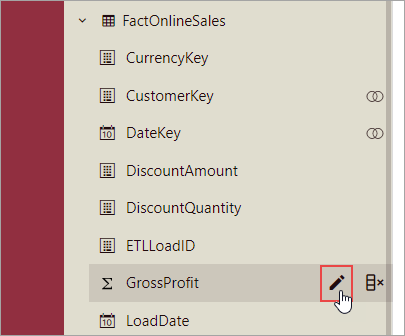4. Enter the new expression or update the existing expression in the editor, and press the Save button.

### To Delete a Measure or a Calculated Column

Follow the below steps to delete a measure or calculated column from an entity.

1. From the Entities panel, select the entity which contains the measure or calculated you no longer require.

2. Then, choose the measure or calculated column.

3. Click the Delete button next to the chosen measure or calculated column.4. In the Delete dialog box that appears, click the Yes button to confirm.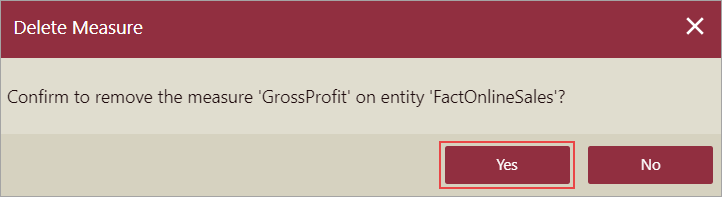### Limitations

1. Preview support is unavailable for measures and calculated columns.

2. Calculated column expressions cannot reference measures, or attributes and expressions referencing other entities.